# Strategies for the Numerical Modelling of Regenerative Pre-heating Systems for Recycled Glass Raw Material

Strategies for the Numerical Modelling of Regenerative Pre-heating Systems for Recycled Glass Raw Material

Carlo CraveroDavide De Domenico Philippe J. Leutcha Davide Marsano

Dipartimento DIME – Università di Genova – via Montallegro 1, 16145 Genova, Italy

Corresponding Author Email:
cravero@unige.it
Page:
324-332
|
DOI:
https://doi.org/10.18280/mmep.060302
20 April 2019
|
Accepted:
10 June 2019
|
Published:
15 September 2019
| Citation

OPEN ACCESS

Abstract:

The rules on energy consumption and pollutant emissions impose increasingly restrictive limitations in today’s industrial sectors. The glass production sector is one of the highest sources of energy consumption in Europe, but also in Italy. For this reason it is very important to develop strategies for consumption reduction in a glass production plant. A glass furnace is in fact conceived with systems to recover heat from the combustion gases through regenerative and recuperative systems in order to increase the efficiency of the plant. Several systems to reduce nitrogen oxides emissions have been also developed and designed. A further method to exploit the residual heat from the exhausted gases is to pre-heat the recycled glass raw material to be introduced into the furnace so as to require a smaller amount of energy for its melting. This paper shows various numerical strategies for the design of a pre-heating system for the recycled glass raw material through CFD techniques. In this regard, numerical models have been developed for systems with direct (hot gases come directly into contact with the raw material) and indirect heat exchange (the raw material is heated through the diffusion of heat from a tube bundle).

Keywords:

glass industry, heat recovery, CFD, numerical optimization

1. Introduction

In this work several numerical approaches for the developing of pre-heating system in a glass production plants have been proposed and developed. This paper is an extended version of a previous paper  where a set of reference CFD models for the pre-heating systems of raw material has been presented.

The glass industry has undergone a strong growth in recent years due to high recyclability of glass material. However, due to the high temperature reached in the furnace, the energy consumption is rather high, and this led to remarkable thermal (CO2) and pollutant emissions (NOx, CO, etc.). It has been estimated that in Italy in 2004 this industrial sector has absorbed about 5% of all industrial energy consumption (1.33 Mtoe) without the addition of the related activities (transport, packaging, etc.).

Due to high temperature reached by the gases flowing from the furnace (around 1400 C), a common strategy to improve glass production plants efficiency provides for the exploitation of the exhaust gases heat through the implementation of heat recovery systems. The most common application consists of regenerative chambers creation, composed of a series of refractory bricks, which cyclically stores the heat and then transfers it to preheat the combustion air. This system has been optimized to reach high efficiency values thanks to several numerical and experimental studies [2-4]. The research team from the University of Genoa has gained relevant experience in the simulation of regenerative glass production plants [5-7], but also in gas recirculation strategies for the reduction of NOx emissions [8, 9].

The gases flowing from the regenerative chamber exit still have, in fact, a considerable amount of energy, with temperatures around 450 C. Since the amount of recycled material inside the glass furnace has become more consistent, the exhaust gases downstream of the regenerative chamber could be further exploited to pre-heat the recycled glass raw material. Similar systems for the pre-heating of raw materials exist in various industrial sectors and have been patented [10-12], such as in steel making [13, 14] in foundries  or for cements . Preheating systems for the glass raw material have already been developed, however there are few numerical models able to model the size of the scrap material and provide an adequate parametric analysis for the design of a pre-heating system. The main target of this paper consists in the development of numerical models for raw material pre-heating systems. First of all, a simplified model, that represents the core of a real pre-heater of recycled glass raw material, has been proposed. This allowed to carry out a sensitivity analysis of the main characteristics of the raw material in order to provide preliminary suggestions for its selection and the consequent thermo-economic analysis of the pre-heater. In fact, through these models the impact of energy consumption savings of the entire glass production plant can be estimated.

Two different methods have been considered: direct and indirect heat exchange. In the former the exhaust gases flow directly into the raw material (throughout a series of holes made on the pipes walls) in the latter the gases flow into pipes without contact with glass (the material is heated only by conduction and thermal radiation). Although the first method is more efficient, due to possible emission problems, some treatment system for the exhausts at the exit of the pre-heater are necessary. The hot gases flowing directly through the raw material can produce some harmful substances. For these reasons the indirect method has been more thoroughly investigated. Furthermore, thanks to CFD calculations, a 0D analytical model has been calibrated; it can be used to quickly drive the design of a pre-heater. Finally, an optimization procedure has been setup; its applicability is demonstrated on a reference design configuration.

2. Applications

In Figure 1 a layout of the typical end-port regenerative furnace is shown with the scheme of the pre-heating system added. The raw material pre-heater is placed downstream the regeneration chamber so the residual heat of the gas can be further exploited. The pre-heater is usually a counter-current tube bundle heat exchanger where the hot gas flow from the bottom upwards and the raw material is fed at the top. The exhaust gases are released into atmosphere by a chimney.

## 1.pngFigure 1. Plant layout with pre-heating system

As previously described both direct and indirect pre-heater systems can be conceived. In the following sections the numerical models developed for the above systems are discussed.

3. Numerical Model

3.1 Granulometry model

The first aspect to consider is the modelling of the size of the material. For simplicity, it has been assumed that the pieces of scrap glass have a parallelepiped shape of size L1×L2×S. The average length defined with the Eq. (1), neglecting the thickness S, is considered:

$L_{m}=\frac{L_{1}+L_{2}}{2}$ (1)

The raw material does not occupy the entire volume inside the pre-heater but a percentage is empty volume. For this reason the parameter Rg is introduced to define the ratio between the volume of glass and the total volume, Eq. (2):

$R_{g}=\frac{v_{g}}{v_{T}}=1-\varepsilon=1-\frac{v_{a}}{v_{T}}$ (2)

Given the size of a scrap of glass (50×50×5 [mm]), the percentage (50 %) of volume that the raw material occupies compared to the total one (Rg), it is possible to derive a relationship between Lm and Rg. This relation has been obtained by performing the regression of the curve passing for the following three points, according to the idea that in a raw material of small size the air percentage is lower than the one of the glass pieces:

• A (Rg;Lm)=(0.5; 50)
• B (Rg;Lm)=(0.01; 300)
• C (Rg;Lm)=(0.99; 1)

The curve reported in the Eq. (3) and in the Figure 2 is obtained:

${{L}_{m}}=a\ln {{R}_{g}}+b(a=-64.67;b=2.568)$ (3)

## 2.png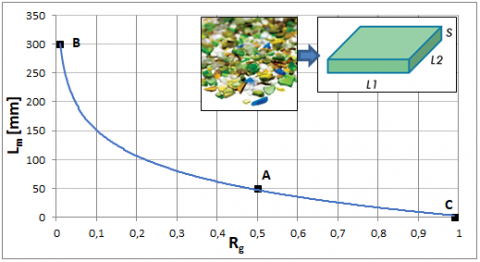Figure 2. Relationship between the glass volume ratio and the size of the raw material

3.2 Indirect method

The preheating system with indirect heat exchange consists of a tube bundle, immersed into the raw material. The hot exhaust gases flow inside the pipes. The heat is transferred by conduction and radiation. In this model the raw material is treated as an equivalent solid composed of air and glass. So appropriate thermo-physical properties have been defined for the equivalent solid. For the equivalent density the mass conservation has been used, Eq. (4):

$\dot{m}_{e q}=\rho_{e q} V_{T}=\dot{m}_{q}+m_{a}=\rho_{p} V_{g}+\rho_{a} V_{a}=\rho_{g}(1-\varepsilon) V_{T}+\rho_{a} V_{a}$ (4)

The equivalent density, and the equivalent conductivity, have been defined as follow:

$\rho_{e q}=(1-\varepsilon) \rho_{g}+\varepsilon \rho_{a}$ (5)

$K_{e \sigma}=(1-\varepsilon) K_{g}+\varepsilon K_{a}$ (6)

The specific heat has been calculated as the weighted average between the specific heat of glass and air, Eq. (7):

$C_{p, e q}=\frac{m_{g}}{m_{e q}} C_{p, g}+\frac{m_{a}}{m_{e q}} C_{p, a} \simeq C_{p, g}$ (7)

where the specific heat of the glass (independent of the raw material size) is dominant.

3.3 Direct method

The preheating system with direct heat exchange consists of a tube bundle with several holes on the tube surface and capped on the upper surface. The exhaust gases flow through the raw material from the pipes holes. The heat exchange takes place by not only conduction and radiation, but also by convection and higher temperatures can be reached in a shorter time. In this case a porous domain has been used, in order to model the volume containing the raw material, with the properties of the glass for the solid part and the properties of the exhaust gases for the fluid part (Cp,f=1290 [J/kgK], Kf=0.0454 [W/mK] and rf =0.53 [kg/m3]). The permeability loss coefficient C1 and the inertial loss coefficient C2 to determine the pressure drop of the exhaust gases in the porous matrix are introduced. One technique for deriving the appropriate constants involves the use of the Ergun equation , applicable over a wide range of Reynolds numbers and for many types of packing. This correlation has been validated in several works dealing with packed bed [19-21], but also by the authors in applications of regenerative chambers [5, 6] and thermal storage CSP . The coefficients C1 and C2 have been defined as follows, Eq. (8-9):

$C_{1}=\frac{1}{\alpha}=\frac{150}{D_{P}^{2}} \frac{(1-\varepsilon)^{2}}{\varepsilon^{3}}$ (8)

$C_{2}=\frac{3.5}{D_{P}} \frac{(1-\varepsilon)}{\varepsilon^{3}}$ (9)

These coefficients depend on the porosity e and on the diameter DP of the equivalent sphere of a piece of raw material that is assumed equal to Lm.

4. CFD Models

Different CFD models have been set up for both direct and indirect heating systems. Using the above models a sensitivity analysis on the properties of the raw material has been performed in order to understand the effects of the main parameters and to derive useful output for design strategies. The CFD analysis have been performed with the Ansys Fluent v.17.1 software, while geometries and grids have been generated with ICEM CFD. The simplified model consists of a representative module of a real pre-heater. Only two pipes, distant 200 [mm] from their centres, constitute the base modulus in order to save computational resources. These pipes are 1.42 [m] height and lay a rectangular base of B=500 [mm] and L=490 [mm]. The tubes have been modelled as smooth walls in the indirect case, because the exhaust gases do not get into direct contact with the raw material. In the direct case a series of small holes has been designed on the side walls of the pipes. Each tube has 52 holes of diameter d=8 [mm] on every 90° arrangement and along the height equally (4×13) distributed; the pipe top is capped. Two different meshes have been generated (Figure 3).

For the indirect case a structured multi-block grid made of O-grid blocks inside the pipes can be generated with about 0.4 Mcells. For the direct case, due to the presence of the holes, an unstructured grid with prism layer has been used with about 7 Mcells. In both cases the first wall cell size gives a Y+ close to 30. The standard k-e with scalable wall functions have been used for the turbulence closure. The transport model of chemical species has been activated for the hot gases that consist of 1 % of Argon, 9.4 % of carbon dioxide, 19% of water vapour and the remaining part of nitrogen. The solver obtained the properties of the gases as the mixing of the individual species properties. Moreover the radiation P1 model is considered. The buoyancy model has been used, in order to consider the free convection motions. The standard 2ND order SIMPLE numerical scheme has been activated. All the simulation performed are unsteady in order to reproduce the thermal response of the system. The time has been fixed to 5 hours to compare the effects of the different solutions. The following boundary conditions have been set: at the inlet a normal massflow rate condition of 0.0288 [kg/s], with a temperature of 730 [K] and a turbulence intensity of 5%; at the outlet an outflow condition has been set. For the pipe wall and the base a no slip condition has been used with a coupled heat transfer condition. The tube is designed as an infinitesimal wall that couples the gas to the raw material, while the thickness (4 [mm]) and the thermal conductivity (15 [W/mK]) are introduced into the numerical interface. A symmetry condition is introduced for the external walls of the box. The small holes in the pipe surfaces for the direct model are treated as interfaces between the fluid and the porous domain.

## 3.pngFigure 3. Mesh cut plane of the two simplified models

5. CFD Analysis

In the following sections several CFD analysis are shown to understand the effects of the main parameters and to compare the different solutions and models. The time history of the average temperature of the raw material volume is used as monitor value to compare.

5.1 Direct model

A parametric analysis has been carried out to gain a sensitivity on the impact of the main parameters on the system response. In the direct model the size of raw material (related to the porosity and consequently the porous resistance) has been varied following Eq. (8-9). Figure 4 compares the volumetric temperature trends over time for different dimensions Lm of the raw material.

## 4.png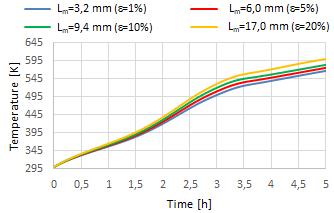Figure 4. Raw material size analysis on direct model

It is clear that as the size of the scrap increases, the system has a faster thermal response. In fact, when the raw material is larger, the void fraction e increases (Fig. 2), the gases can find the way through the packed bed more easily and therefore the convection heat exchange is enhanced. A variation from e=0.1 to 0.2 gives about 5 [K] difference while a negligible temperature change is observed in the range e=0.01 - 0.1.

5.2 Indirect model

The comparison of volumetric raw material thermal response with the different pre-heating systems (direct and indirect) has been reported in Figure 6. The same glass and dimension properties has been adopted for the two cases.

## 5.pngFigure 5. Comparison between the indirect and direct CFD model – thermal response

## 6.png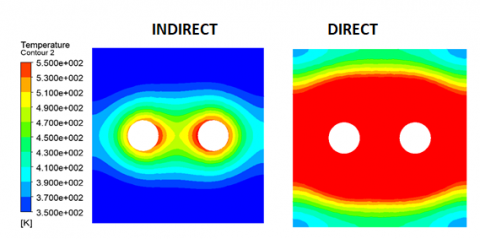Figure 6. Comparison of the temperature contours of the glass raw material between the indirect and direct method (midspan section)

It is clear how, in the same conditions, it is possible to obtain a temperature value higher than 200 [K] in the direct case at the end of the pre-heating time. This difference has been also observed in the temperature field in the contours of Figure 6 for the direct and indirect case.

The direct method is clearly more efficient than the indirect case. However, since the direct method requires appropriate filtering systems for the gases which can release harmful substances and powder, it requires special post treatment systems and it is therefore less attractive. For this reason the attention has been focused on the indirect approach in order to develop models and tools for its optimal design. In order to make the comparisons easier a baseline case has been identified (Table 1).

Table 1. Glass baseline case properties

 e Cp,g [J/kgK] Kg [W/mK] rg [kg/m3] αg [mm2/s] 0.05 800 0.75 2575 0.364

Attention has been given to the individual properties of the scrap glass and its size. An equivalent thermal diffusivity, defined as in the Eq. 10-11, is introduced to compare different combinations of material properties and size.

$\alpha_{e q}=(1-\varepsilon) \alpha_{q}+\varepsilon \alpha_{a}$ (10)

$\alpha \text{=}\frac{k}{\rho {{C}_{p}}}$ (11)

In Table 2 the set of cases with different equivalent thermal diffusivity are described. In C1, C2 and C3 the thermal diffusivity of the glass has been changed keeping the same size of the raw material (same e); in the other three cases the size of the scrap (different e) has been varied with constant glass properties.

Table 2. Set of cases having different equivalent thermal diffusivity with respect to baseline

 Case αg [mm2/s] αa [mm2/s] e αeq [mm2/s] C1 0.291 21.9 0.05 1.373 C2 0.324 21.9 0.05 1.404 C3 0.413 21.9 0.05 1.488 C4 0.364 21.9 0.01 0.580 C5 0.364 21.9 0.1 2.520 C6 0.364 21.9 0.2 4.676

The system response by changing the thermal diffusivity of the glass is shown in Figure 7, while in Figure 8 have been reported the thermal response by changing the size of the raw material. As the equivalent thermal diffusivity increases, a faster system response can be obtained. Figure 7 shows that a raw material with a higher thermal conductivity, lower specific heat and density, is more advantageous. In fact a difference of 15 [K] between case C1 and C3 has been identified at the end of the preheating time. Figure 8 shows that a bigger raw material provides a faster thermal response, in a similar way to the direct case (Figure 4). However it is to be noticed that there is a less marked variation (less than 10 [K]) between cases C4 and C6 at the end of the preheating time with respect the analysis with different properties of the glass.

## 7.pngFigure 7. Glass thermal diffusivity analysis on indirect model

## 8.pngFigure 8. Raw material size analysis on indirect model

5.3 Energetic considerations

The results of the previous parametric analysis on the impact of size and properties of the scrap material can be used to estimate the variation in the consumption of the glass production plan. In fact, a designer through the energy balance can calculate the fuel savings necessary for the glass furnace, considering the temperature change of the recycled material at the end of the preheating time. The heat flux that the fuel (methane) has to provide to the glass bath following the observed temperature change compared to the baseline case, at the end of the pre-heating time is:

$\dot{m}_{g} C_{p, g} \Delta T_{b a s e l i n e}=\Delta q=\Delta \dot{m}_{C \Pi_{4}} H_{i}$ (12)

Assuming that in a generic glass furnace 356 tons/day (or 4.12 [kg/s]) of raw material to melt are introduced and that a generic glass bath absorbs 8.3 [MW] , the percentage variations of the fuel massflow rate can be predicted; consequently the economical effects on the furnace management can be estimated. Assuming that the percentage of recycled material is in the range 20 and 80 %, the impact on fuel is predicted accordingly in Table 3, for all the cases previously simulated. The change in fuel percentage with the minus sign represents a savings; the positive sign in the variation means higher fuel consumption.

From this analysis it is clear that raw material with high equivalent thermal diffusivity, is preferable. With the estimated fuel consumption from an average furnace  giving an annual cost of the order 14 M€, a cost increase of 70 k€ with respect to baseline solution is expected with solution C1.

Table 3. Fuel percentage variation for the several cases

 Case $\Delta {{\dot{m}}_{C{{H}_{4}}}}$  [%] C1 (0.12÷0.48) C2 (0.06÷0.24) C3 - (0.03÷0.10) C4 (0.01÷0.05) C5 -(0.02÷0.06) C6 -(0.06÷0.22)
6. Analitical Model

This section describes an analytical model based on the lumped parameters approach developed for a quick analysis of raw material pre-heating system in the indirect heat transfer case. The results of the analytical model are compared to those from CFD in order to tune the 0D approach. To calculate the heating rate of the raw material, the energy balance is imposed, Eq. (13). The internal energy variation of the raw glass is equal to the variation of the thermal heat flow through the system. The incoming heat flow includes the heat entering from the pipes, Eq. (14), and the heat entering from the lower and upper base of the box; the heat dispersed in the environment is clearly a loss, Eq. (15).

$\rho_{g} V_{g} C_{p, g} \frac{\delta T_{g}}{\delta t}=\left(q_{\text {nibes }}+q_{\text {bottom }}+q_{\text {top }}\right)=q_{\text {loss}}$ (13)

## 14.png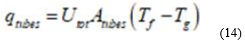## 15.png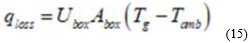The upper and lower base have been neglected. The average dispersion efficiency has been introduced, Eq. (16). In this way Eq. (13) can be simplified in Eq. (17).

## 16-17.pngThe analytical solution of the previous equation is reported in the Eq. (18). It gives the temperature trend of the equivalent solid over time, where the exponent is the ratio between the time and the characteristic time of the system, as reported in Eq. (19).

## 18-19.png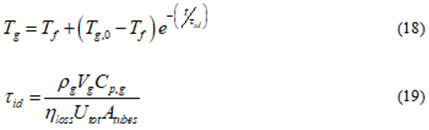The characteristic time depends on the thermal inertia of the equivalent solid, on the tube exchange surface, on the box dispersion efficiency and finally on the transmittance (Eq. 20), that considers the conductive resistance of the tube and the internal convective resistance.

## 20.png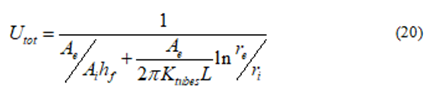Forced convection in pipes is described by the following dimensionless parameters: Reynolds number, Eq. (21), Prandtl number, Eq. (22) and Nusselt, Eq. (23).

$R e_{D}=\frac{\rho_{f} v_{f} D}{\mu_{f}}$ (21)

$\operatorname{Pr}=\frac{\mu_{f} C_{p, f}}{K_{f}}$ (22)

$N u_{x}=\frac{h_{f} D}{K_{f}}=f\left(R e_{D}, P r\right)$ (23)

As the flow regime inside the tubes is similar to a not fully developed motion, the correlations for the calculation of the local Nusselt number have been reported in Eq. (24), the first line is valid when ${{x}_{*}}\le 0.01$ , while the second when  ${{x}_{*}}$>0.01. This regime is valid if the dimensionless abscissa, Eq. (25), respects the condition reported in Eq. (26) at the exit of the tube.

$N u_{x}=\left\{\begin{array}{c}{1.077 x_{*}^{-1 / 3}-0.7} \\ {3.657+6.874\left(x_{ .} \times 10^{3}\right)^{-0.488} e^{-57.2 x_{ .}}}\end{array}\right.$ (24)

$x_{*}=\frac{x / D}{R e_{D} P r}$ (25)

$x_{*}(H)<\overline{x}_{*}=0.05$ (26)

This analytical 0D model has been applied to three different sizes of raw material (different e). In Figure 9 the temperature distributions are compared to those obtained by the CFD.

## 9.pngFigure 9. Comparison between 0D and CFD models

## 10.png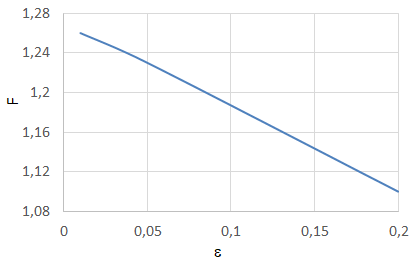Figure 10. Correlation factor vs size of raw material

Using the above curves a calibration factor for the 0D analytical model can be obtained. The characteristic time from CFD results has been estimated. Table 4 shows the numerical values of the calibration factors F defined as the ratio $\frac{{{U}_{tot,CFD}}}{{{U}_{tot,0D}}}$ for different size of raw material (e). Figure 10 shows the relationship between the calibration factor and the size of the raw material.

The above relation is introduced to use the 0D model for quick parametric analysis for the preliminary design phases before a CFD simulation campaign.

Table 4. Calibration factors for different size of raw material

 e F 0.01 1.26 0.05 1.23 0.2 1.1
7. Design Optimization Procedure

A design optimization strategy based on the response surface approach has been developed. This approach has been used for several different applications and it is very effective when the numerical simulation of the system is computationally expensive of if many design variables are considered . The goal of this optimization procedure is to obtain the pipe geometry (thickness and diameter) that maximizes the final temperature of the raw material and minimizes the mass flow rate of the hot gases. A Design of Experiment (DOE) dataset of configurations has been generated with the Latin Hypercube Sampling model. The range of the parameters that define the design space is reported in Table 5. A DOE with 80 samples has been generated and validated. In Figure 11 the obtained mass flow rate from each CFD simulation (using the same CFD model and settings for indirect heat transfer previously described) of the DOE is reported (blue dots). In the same figure, the individuals generated by the optimization process (using the response surface) are also reported (red dots).

Table 5. Parameter ranges for the optimization procedure

 D [mm] S [mm] ṁ [kg/s] 80÷100 1÷5 0.01÷0.02

## 11.pngFigure 11. DOE and MOGA individuals for mass flow rate

Table 6. Geometrical data of the optimized case (for the simplified model)

 D [mm] S [mm] ṁ [kg/s] 91.63 3.8 0.0162

## 12.pngFigure 12. Pareto front

## 13.pngFigure 13. Optimized vs baseline case temperature trends

The Dakota software platform has been used for the optimization procedure. The multi-objective genetic algorithm MOGA has been used for the optimization process working on the response surfaces of the mass flow rate and of the final temperature from the heating. After a sensitivity analysis over the number of populations and generations, the MOGA has been set up with a total of 12.000 iterations to play on the response surfaces The Pareto Front obtained is shown in Figure 12. The optimum point on the Pareto Set of Figure 12 that has a lower mass flow and a higher final temperature than the reference case has been selected. The corresponding geometrical data of the optimized case are reported in Table 6.

In order to validate the optimization procedure, a CFD simulation has been performed on a complete geometry composed of 15 tubes that is a reference geometry for a case under study . The results of the optimized case, based on one pipe model, have been compared with the baseline case with the complete geometry. Figure 13 shows the different temperature trends of the original and optimized configuration. In Figure 14 the temperature distributions on a transverse plane at midspan show the higher heat exchange effect on the optimized case.

## 14.pngFigure 14. Comparison of the temperature contours of the glass raw material between the baseline and the optimized case (midspan section)

The results confirm the improvement in the optimised configuration with respect to baseline. A reduced massflow rate of 0.6 % and a lighter pipe (larger diameter, and lower thickness), give a higher raw material temperature of over 25 [K]. It results in a saving of fuel for the glass furnace, but also in lower costs for the constructions of the pre-heater due to the reduction of steel needed.

8. Conclusions

Several models for the pre-heating of raw material based on a representative module have been presented. Two different pre-heating systems (direct and indirect) have been considered, but more attention has been given to the second method; in fact, despite its lower efficiency, it does not require any special exit gas treatment. During the design of a pre-heater, these models can estimate the main effects based on the raw material properties. In fact, a parametric analysis has been carried out to consider different size of the raw material and its main thermal properties (grouped in the equivalent thermal diffusivity). Through this analysis it is also possible to evaluate the energetic impact on the glass furnace and consequently the economic effects. A 0D analytical model has been calibrated in order to provide a quick reference design tool to understand the main effects of the design parameters.

Finally an optimization procedure has been carried out on a simplified two pipe model. This procedure has been tested on a real case with 15 tubes to show how its effectiveness. In the optimized case, a lower mass flow rate of exhaust gases and a reduced weight of the pipes give a faster thermal response and lower costs.

Acknowledgment

The present work has been supported by a funding from Regione Liguria POR FESR Liguria 2014-2020 – Asse 1 – Azione 1.2.4 CUP G38I17000010007 to support industrial research and innovation.

Nomenclature
 A area, [m2] B base length, [m] C1 permeability loss coefficient, [1/m2] C2 inertial loss coefficient, [1/m] Cp specific heat, [J/kgK] d hole diameter, [mm] D diameter, [mm] Dp mean particle diameter, [m] F calibration factor h convective heat transfer coefficient, [W/m2K] H height, [m] Hi lower heating value, [J/kg] K thermal conductivity, [W/mK] L width, [m] Lm average length of the raw material, [mm] m mass, [kg] ṁ massflow rate, [kg/s] Nu Nusselt number Pr Prandtl number q heat, [W] r radius [mm] Re Reynolds number Rg glass ratio S thickness, [mm] t time, [s] T temperature, [K] U transmittance, [W/m2K] v velocity, [m/s] V volume, [m3] x local coordinate, [m] Y+ non dimensional boundary layer distance from wall Greek symbols α thermal diffusivity, [mm2/s] e porosity η efficiency µ dynamic viscosity, [Pa s] r density, [kg/m3] τid characteristic time, [s] Subscript 0 initial condition a air amb ambient e external eq equivalent f exhaust gas g glass i internal T total
References

 Cravero, C., Leutcha, P.J., Marsano, D. (2019). CFD modelling of regenerative pre-heating systems for recycled glass raw material. TECNICA ITALIANA-Italian Journal of Engineering Science, 63(2-4): 189-197. https://doi.org/10.18280/ti-ijes.632-411

 Zarrinehkafsh, M.T., Sadrameli, S.M. (2004). Simulation of fixed bed regenerative heat exchangers for flue gas heat recovery. App. Therm. Eng., 24(2-30: 373-382. https://doi.org/10.1016/j.applthermaleng.2003.08.005

 Reboussin, Y., Fourmigué, J.F., Marthy, J.F., Citti, O. (2005). A numerical approach for the study of glass furnace regenerators. App. Therm. Eng., 25(14-15): 2299-2320. https://doi.org/10.1016/j.applthermaleng.2004.12.012

 Koshelnik, A.V. (2008). Modelling operation of system of recuperative heat exchangers for aero angine with combined use of porosity model and thermos-mechanical model. Glass and Ceramics, 65(9-10): 301-304.

 Basso, D., Cravero, C., Reverberi, A.P., Fabiano, B. (2015). CFD analysis of regenerative chambers for energy efficiency improvement in glass production plants. Energies, 8: 8945-8961. https://doi.org/10.3390/en8088945

 Cravero, C., Marsano, D. (2017). Numerical simulation of regenerative chambers for glass production plants with a non-equilibrium heat transfer model. WSEAS Transactions on Heat and Mass Transfer, 12(Art. 3): 21-29.

 Cravero, C., Marsano, D., Spoladore, A. (2017). Numerical strategies for fluid-dynamic and heat transfer simulation for regenerative chambers in glass production plants. NAUN International Journal of Mathematical Models and Methods in Applied Sciences, 11: 82-87.

 Cogliandro, S., Cravero, C., Marini, M., Spoladore, A. (2017). Simulation strategies for regenerative chambers in glass production plants with strategic exhaust gas recirculation system. 11th AIGE 2017, 2nd AIGE/IEETA Int. Conf., 12-13 June 2017, Genova, Italy – International Journal of Heat and Technology, ISSN: 0392-8764, 35(Sp1):  S449-S445. https://doi.org/10.18280/ijht.35Sp0161

 Cravero, C., De Domenico, D. (2019). The use of CFD for the design and development of innovative configurations in regenerative glass production furnaces. Energies, 12(13): 2455. https://doi.org/10.3390/en12132455

 Sweo, B.J., Ginther, J.H. (1965). Method for raw material pre-heating for glass melting. U.S. Patent 3 185 554, May 25, 1965.

 Nesbitt, J.D., Fejer, M.E. (1974). Process for pre-treating and melting glassmaking materials. U.S. Patent 3 788 832, Jan. 29, 1974.

 Suzuki, T., Murao, M., Utiyama, S., Hatanaka, K., Inoue, H. (1979). Premelting method for raw materials for glass and apparatus relevant thereto. U.S. Patent 4 135 904, Jan. 23, 1979.

 Nakano, H, Uchida, S., Arita, K. (1999). New Scrap Preheating System for Elecrtic Arc Furnace (UL-BA). Nippon Steel Technical Report No. 79, 1999.

 Mandal, K. (2010). Modeling of scrap heating by burners. PhD Thesis, Department of Materials Science and Engineering, McMaster University, Hamilton, Ontario, Canada.

 Pariona, M.M., Mossi, A.C. (2005). Numerical simulation of heat transfer during the solidification of pure iron in sand and mullite molds. J. of the Braz. Soc. Of Mech. Sci. & Eng., XXVII(4): 399-406. https://doi.org/10.1590/S1678-58782005000400008

 Mujumdar, K.S., Ganesh, K.V., Kulkarni, S.B., Ranade, V.V. (2007). Rotary Cement Kiln Simulator (RoCKS): Integrated modeling of pre-heater, calciner, kiln and clinker cooler. Chemical Engineering Science, 62(9): 2590-2607.  https://doi.org/10.1016/j.ces.2007.01.063

 Cravero, C., Leutcha, P., Mola, A., Nilberto, A., Spoladore, A. Experimental and numerical investigations on pre-heating systems for recycled glass in glass production plants.

 Ergun, S. (1952). Fluid flow through packed columns. Chem. Eng. Prog., 48(2): 89-94.

 Xu, Z., Woche, H., Specht, E. (2009). CFD flow simulation of structured packed bed reactors with jet injections. The 14TH International Conference on Fluid Flow Technologies, Budapest, Hungary, pp. 536-544.

 Mohammadpour, K., Woche, H., Specht, E. (2017). CFD simulation of parallel flow mixing in a packed bed using porous media model and experiment validation. Journal of Chemical Technology and Metallurgy, 52(3): 475-484. https://doi.org/10.1007/s40571-018-0203-x

 Faruoli, M., Viggiano, A., Magi, V. (2019). c. TECNICA ITALIANA-Italian Journal of Engineering Science, 63(2-4): 291-296.

 Mahmood, M., Traverso, A., Traverso, A.N., Massardo, A.F., Marsano, D., Cravero, C. (2018). Thermal energy storage for CSP hybrid gas turbine systems: Dynamic modelling and experimental validation. Applied Energy, 212: 1240-1251. https://doi.org/10.1016/j.apenergy.2017.12.130

 Madivate, C., Muller, F., Wilsmann, W. (1998). Calculation of the theoretical energy requirement for melting technical silicate glasses. Journal of the American Ceramic Society, 81(12): 3300-3306. https://doi.org/10.1111/j.1151-2916.1998.tb02771.x

 Cravero, C., Macelloni, P., Briasco, G. (2012). Three-dimensional design optimization of multi stage axial flow turbines using a RSM based approach. Asme paper GT2012-68040, Asme Turbo Expo, Copenhagen (DK), June 11-5, 2012.